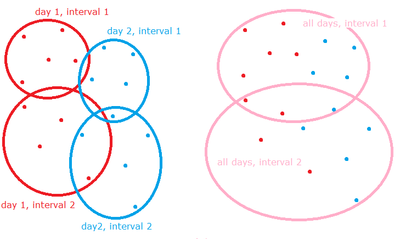# Generate hourly perimeters from daily data

202
5
10-10-2021 09:32 PM
Labels (1)by
New Contributor II

Hi all,

I have spatial-temporal point data. The point data represents locations at various times over the course of a couple of days. I would like to predict locations at fixed hourly intervals within a day (e.g every hour or every 4 hours) as polygons. All research has been leading back to the idea of using interpolation but I'm unsure of how to maneuver the tool for this purpose. Any recommendations on how to go about this?

Thanks for any input!

Best,

Rachel

5 RepliesbyMVP Regular Contributor

Couldn't you just use the convex hull of all points in the same interval?

``````import datetime
# variables
points_fc = "PointFC"
date_field = "DateField"
time_interval_minutes = 60
polygon_buffer = 100

# read shape and date
data = [r for r in arcpy.da.SearchCursor(points_fc, ["SHAPE@", date_field], sql_clause=(None, "ORDER BY " + date_field))]
# construct time bins
start_time = data
end_time = data[-1]
time_bins = [start_time]
while time_bins[-1] < end_time:
time_bins.append(time_bins[-1] + datetime.timedelta(minutes=time_interval_minutes))
# create output fc
sr = arcpy.Describe(points_fc).spatialReference
polygons = arcpy.management.CreateFeatureclass("memory", "polygons", "POLYGON", spatial_reference=sr)
# fill output fc
with arcpy.da.InsertCursor(polygons, ["SHAPE@", "StartTime", "PointCount"]) as cursor:
for b in range(len(time_bins)-1):
# get points that belong to the interval
t0 = time_bins[b]
t1 = time_bins[b+1]
points = [d.firstPoint for d in data if d >= t0 and d < t1]
# if there are points in the interval
if points:
# change list of point geometries to multipoint geometry
multipoint = arcpy.Multipoint(arcpy.Array(points))
# get the convex hull, buffer to prettify
polygon = multipoint.convexHull().buffer(polygon_buffer)
# insert
cursor.insertRow([polygon, t0, len(points)])``````

Have a great day!
JohannesbyMVP Regular Contributor

I think I misunderstood your question...

The code above shows you the locations in a certain time interval. You want to know the probable locations in a time interval during a random day. Basic idea still applies:

``````from datetime import date, time, timedelta
# variables
points_fc = "PointFC"
date_field = "DateField"
time_interval_minutes = 60
polygon_buffer = 100

# read shape and date
data = [list(r) for r in arcpy.da.SearchCursor(points_fc, ["SHAPE@", date_field])]
for d in data:
d.append(datetime.combine(date.today(), d.time()))
# construct time bins
start_time = datetime.combine(date.today(), time(0, 0))
end_time = start_time + timedelta(days=1)
time_bins = [start_time]
while time_bins[-1] < end_time:
time_bins.append(time_bins[-1] + timedelta(minutes=time_interval_minutes))
# create output fc
sr = arcpy.Describe(points_fc).spatialReference
polygons = arcpy.management.CreateFeatureclass("memory", "polygons", "POLYGON", spatial_reference=sr)
# fill output fc
with arcpy.da.InsertCursor(polygons, ["SHAPE@", "StartTime", "PointCount"]) as cursor:
for b in range(len(time_bins)-1):
# get points that belong to the interval
t0 = time_bins[b]
t1 = time_bins[b+1]
points = [d.firstPoint for d in data if d >= t0 and d < t1]
# if there are points in the interval
if points:
# change list of point geometries to multipoint geometry
multipoint = arcpy.Multipoint(arcpy.Array(points))
# get the convex hull, buffer to prettify
polygon = multipoint.convexHull().buffer(polygon_buffer)
# insert
cursor.insertRow([polygon, t0.strftime("%H:%M"), len(points)])``````

Have a great day!
Johannesby
New Contributor II

Johannes,

Thank you for taking the time to assist. I understand that you described that the solution would generate polygons representing locations in a time interval during a random day. May you clarify if it could generate a feature class representing sub-daily time intervals from the daily data? I want to examine time intervals within the course of time that the data takes place (e.g. dataset took place over a 3-day time period and I want values for every 4 hours within those 3 days).byMVP Regular Contributor

Sorry, I find it hard to describe, as English isn't my first language. Hopefully the illustration helps...

Imagine you have location data over two days (red and blue points).

The first code sample will give you the polygons on the left side: one polygon for each time interval on each day. "Where were you on day 1, interval 2?"

The second code sample will give you the polygons on the right side: one polygon for each time interval on all observed days. "Where were you on any day, interval 2?"Have a great day!
Johannesby
New Contributor II

The illustration was very helpful. I'll give it a try.